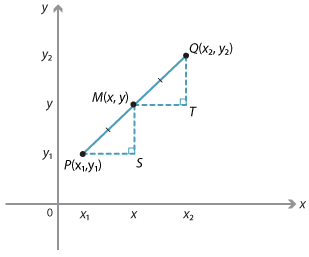#### General caseDetailed description

We can find a formula for the midpoint of any interval. Suppose that $$P(x_1, y_1)$$ and $$Q(x_2, y_2)$$ are two points and let $$M(x, y)$$ be the midpoint.

Triangles PMS and MQT are congruent triangles (AAS),
and so $$PS = MT$$ and $$MS = QT$$.

Hence the x-coordinate of M is the average of $$x_1$$ and $$x_2$$, and the y-coordinate of M is the average of $$y_1$$ and $$y_2$$. Therefore:

$$x = \dfrac{x_1 + x_2}{2} \text{and}\ y = \dfrac{y_1 + y_2}{2}$$.